﻿ Modelling Plant Growth Based on Gompertz, Logistic Curve, Extreme Gradient Boosting and Light Gradient Boosting Models Using High Dimensional Image Derived Maize (<i>Zea mays</i> L.) Phenomic DataPublications are Open
Access in this journal
Article Versions
Export Article
• Normal Style
• MLA Style
• APA Style
• Chicago Style
Open Access Peer-reviewed

### Modelling Plant Growth Based on Gompertz, Logistic Curve, Extreme Gradient Boosting and Light Gradient Boosting Models Using High Dimensional Image Derived Maize (Zea mays L.) Phenomic Data

Peter Gachoki, Moses Muraya, Gladys Njoroge
American Journal of Applied Mathematics and Statistics. 2022, 10(2), 52-64. DOI: 10.12691/ajams-10-2-3
Received July 13, 2022; Revised August 19, 2022; Accepted August 28, 2022

### Abstract

Modelling of plant growth is vital for hypotheses testing and carrying out virtual plant growth and development experiments, which may otherwise take a long time under field conditions. Modelling of plant growth has been aggravated by new phenotyping platforms that generate high dimensional data non-destructively over the entire growth time of a plant using a set of camera system. Such platforms generate high-throughput phenomic data, which is complex and constitute many features collected at multiple growth points for the same plant. However, the classical models are limited in that they can only model a single feature at a time. The objective of this study was to apply dynamic plant growth models that could be used to dissect complex relationships between plant growth and development using several modelling strategies. These included sigmoid, light GBM and XGBoost models. The image derived phenomic data was obtained from the Leibniz Institute of Plant Genetics and Crop Plant Research Gatersleben, Germany. The models were fitted using R statistical software and compared based on RMSE, R-squared, AIC and BIC performance metrics. The results showed that the XGBoost (RMSE = 2.1641) and Light GBM (RMSE = 2.7776) performed better than the Gompertz (RMSE = 3.8378) and the logistic function (RMSE = 3.8378) models in modelling maize plant growth. The XGBoost model (RMSE = 2.1641) showed better performance than Light GBM model (RMSE = 2.7776) in modelling maize plant growth. The Gompertz model using plant volume had AIC and BIC values for 139738.3 and 139763.4, respectively. The Gompertz model for plant side area had AIC and BIC values for 98436.15 and 98461.31, respectively. The logistic function model for plant volume had AIC and BIC values for 139749.2 and 139774.4, respectively. The logistic function model for plant side area had AIC and BIC values for 98415.95 and 98441.11, respectively. The Gompertz model and logistic function models showed almost the same performance in modelling maize plant growth. The non-parametric models, the XGBoost and light GBM, were found to perform better than the classical models (Gompertz and logistic functions) in modelling maize plant growth. Therefore, the study recommends the use of XGBoost as a generic model to fit high dimensional and complex phenotypic data in modelling plant growth and prediction of plant biomass yield at different growth points.

### 1. Introduction

Modelling plant growth enables scientists and researchers to test theories and do virtual trials concerning plant growth and development that could have otherwise taken long time under field conditions 1. The statistical models allow for interpretation of complex regulatory processes contributing to plant growth and development. Such models are informative instrument that are helpful in research 2. In addition, growth models help investigators to systematically analyse systems perturbations, develop hypotheses to guide the design of new experimental tests, and ultimately assess the suitability of specific novel traits.

Modelling of plant growth has become a key research area especially in the field of agriculture, environmental science and forestry 3. This has been facilitated by the sharing of resources and experiences between the mathematicians, biologists and computer scientists. This modelling of plant growth through integration of knowledge from various disciplines is necessary for advanced research in plant development and growth as well as in simulation studies 3. Modelling of plant growth such as maize can be important in improving production and yield. This is because modelling can help to understand the dynamics of plant growth and development, and yield responses under different growth conditions 4.

The use of plant growth models to assess genotypes performance across diverse target environments, can help understand suitability of trait variation, and possibly accelerate plant breeding programs. However, the models to support these tasks are still under development and testing. Moreover, currently the phenotyping methods have changed, including use large-scale imaging phenotyping, which derive large, high dimensional and complex data set on all aspect of plant growth and development 5, 6, 7, 8. Such large and high dimensional data may not be appropriately fitted by existing models 9. Therefore, the existing model may require an enabling data inference technology in addition to algorithm development with the aim of analytically solving complex and high dimensional data sets 10, 11. Phenotyping has advanced with the application of high throughput phenotyping such as use of automated imaging techniques 8. This has led to derivation of large quantities of high dimensional data or phenotypic features that could not have been achieved using manual phenotyping in a single run 7. This called for parallel development of statistical models that can appropriately handle such high dimensional data. Information on such models is limited. Though existing models such as the sigmoid models have been found to predict plant growth with high degree of precision, information on their performance using high dimensional data was still limited.

Models exist that have been used in modelling plant growth and development. However, such models have been used to fit low dimensional data derived using manual measurement 3, 12. The manually collected is limited to few features and the same plant cannot be measured in subsequent growth stages since the plant is destroyed during the data collection. Hence, such models may be inefficient in fitting the high dimensional data that is derived using high-throughput techniques and at different growth stages for the same plant. Some of the existing models for plant growth include the sigmoid models, simple linear regression models, multiple linear regression models and non-linear models such as the quadratic and the cubic models.

Sigmoid models are better suited to individual plant growth and development 5. These models include the exponential and monomolecular models 13. These models have been found useful in interpreting individual growth patterns 3. Linear models have also been developed by expressing the dry weight of the shoot as a function of plant area and plant age 14. Using the same variables, quadratic and cubic models were also with the linear regression model showing better performance as compared to the fitted non-linear models 14. Moreover, simple linear regression and multiple linear regression models had been used to model growth and good performance has been exhibited 5, 15.

Despite good performance of the existing growth models, it is worth noting that the models can only accommodate a few number of features. Therefore, the is need to test the performance of these models in case of data with high dimensionality derived using automated phenotyping platforms, such as image derived data. The data from automated phenotyping platforms are quite noisy 16. Moreover, this high dimensional data has many features, which can perhaps aid in coming up with a more actuality plant growth curve and thus improved prediction. Fitting such data set using the existing classical models, may lead to over fitted models thus affecting their prediction accuracy. Therefore, alongside the already existing models, there is also need to test how non-parametric models such as the XGBoost and light GBM would fit the high dimensional data. Since these non-parametric models had not been used in modelling plant growth 17, there is need to compare their prediction accuracies with the already existing plant growth models.

A study by 18 showed that non-parametric machine learning models handle more data than the other statistical models. This is an important strength that is really useful in handling large dimensional high-throughput data. Some of the most effective predictive models, such as XGBoost and Light GBM continue to be accurate with thousands or millions of additional data features 18, 19, 20. Additionally, the non-parametric models have the mechanisms to sort out the variables that contain information relevant to the outcome and those variables that would just add noise to the predictions 21. Generally, the conventional models that have been so far been applied for growth modeling don’t have such inbuilt mechanisms. In extreme situations where the predictor variables are more than the observations, these models results to total failure 22. In addition, for the existing growth models, the data must meet some underlying assumptions failure to which the prediction accuracy will be low 22.

The existing models needed to be tested alongside newly promising models such as extreme gradient boosting and light gradient boosting. The modelling of plant growth was a regression problem and thus the existing models were compared based on akaike information criterion (AIC), Bayesian information criterion (BIC), root mean squared error (RMSE), mean absolute error (MAE) and r-squared 23. Smaller values of AIC, BIC, RMSE and MAE shows that a model is a better fit. Larger values of r-squared is an indication that a model is a better fit 23. For instance, 24 compared the performance of non-linear mathematical models in describing growth. The criterions used were; the coefficient of determination, Akaike information criterion, root mean squared error and Bayesian information criterion. The models fitted were; Richards, Gompertz and logistic model for different animals 24. The results showed that the Richards models provided a better fit to the experimental data. It was also noted that the different models showed better performance for some animals. In a study that fitted growth models, the models were compared using the Akaike information criterion and the Bayesian information criterion 25. The models fitted were; log logistic, weibull, exponential, logistic and Gompertz models. The best models were the logistic and the log logistic.

### 2. Methodology

The data was obtained from Leibniz Institute of Plant Genetic and Plant Crop Research (IPK-Gaterleben), Gatersleben, Germany. All the analysis was carried out using the R statistical software. The features that used in model fitting were as described in 26. The features were selected using feature importance statistical technique based on their predictability of the manually collected plant biomass (Table 1).

The selected features (Table 1) were used to fit the logistic curve, the Gompertz model, XGBoost and Light GBM models. A comparison was then made using RMSE, R-squared, AIC and BIC on how the non-parametric models and sigmoid models performed in modelling plant growth and biomass prediction.

2.1. Logistic Growth Curve

Logistic growth curve is an S-shaped (sigmoidal) curve that can be used to model functions that increase gradually at first, more rapidly in the middle growth period, and slowly at the end, levelling off at a maximum value after some period of time 27. Two models of the logistic growth curve were fitted in this study. The first logistic curve model had the equation;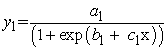where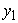= volume,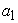= the asymptote (the maximum volume that could be attained),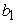= the displacement on the x-axis,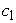= the growth parameter (described how quickly the variableapproached the asymptote).

The second logistic curve model had the equation;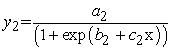where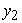= side area,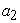= the asymptote (the maximum side area that could be attained),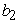= the displacement on the x-axis,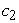= the growth parameter (described how quickly the variableapproached the asymptote).

2.2. Gompertz Growth Model

Gompertz model is a sigmoid model that is frequently used with growth data and other data. Two Gompertz models were fitted in this study. The first Gompertz model had the equation;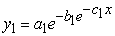where= variable volume,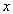= time,= the asymptote or the carrying capacity obtained by doing the limit of the function when volume tends to infinity (the highest volume that could be attained),= the displacement on the x-axis,= the growth rate.

The second Gompertz model had the equation;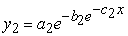where= side area,= time,= the asymptote or the carrying capacity obtained by doing the limit of the function when side area tends to infinity (the highest side area that could be attained),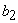= the displacement on the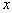-axis,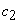= the growth rate.

2.3. Extreme Gradient Boosting Model

Extreme Gradient Boosting (XGBoost) is a tree-based algorithm, which sits under the supervised branch of Machine Learning. In its development, new training data sets were formed by random sampling with replacement from the original dataset, during which some observations were repeated in each new training data set. The observations were weighted and therefore some of them were selected in the new datasets more often. The XGBoost models fitted for this regression problem had the following general form.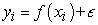where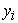= variable being predicted at a specific time point (plant biomass),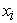= phentotypic features at the specific time point such as; side area, side length, leaf length, top area, side border length, top hull area, side height, volume, side leaf length, side leaf width and top leaf width (Table 1).

The training data was divided into subsets and the final prediction was a weighted sum of all the decision tree functions. In fitting the XGBoost models, samples were selected sequentially. For instance, the first sample was selected and a decision tree was fitted. The model then picked the examples that were hard to learn and using them and a few others selected at random from the training data set, a second model was fitted. Prediction was then made using the first and the second models. The model was then evaluated and hard examples were picked together with other randomly selected examples from training set and another model was fitted. The process of boosting algorithms continued up to a number 𝑛. In other words, the first model is fitted using the original training set. For example, a simple regression model;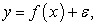if the error was too large, the solution could have been to add more features, use another algorithm, tune the algorithm or look for more training set. However, if the error was not white noise and it had a relationship with the output, the second model was fitted as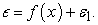The process continued n-times and the final model was;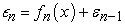The final step involved adding these models together with some weighing criteria where the weights =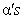which yielded the final function that was used for prediction.

2.4. Light GBM Model

In Light GBM, the base learners were generated sequentially in such a way that the present base learner was always more effective than the previous one. This kind of boosting tried to optimize the loss function of the previous learners by a new adaptive model that added weak learners in order to reduce the loss function. The Light GBM models fitted for this regression problem had the following general form.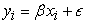where= variable being predicted at a specific time point (plant biomass),= phentotypic features at the specific time point such as; side area, side length, leaf length, top area, side border length, top hull area, side height, volume, side leaf length, side leaf width and top leaf width (Table 1). The fitting process of the Light GBM model was similar to the one outlined in section 2.3.

2.5. Models Validation and Comparison

The models were validated by using the cross validation statistical methods. In cross-validation, models are evaluated by training several models on subsets of the available input data and evaluating them on the complementary subset of the data. In this study 10-fold cross-validation method was used. In the 10-fold cross-validation, the input data was split into 10 subsets of data. The models were trained on all but one (10 - 1) of the subsets, and then evaluated on the subset that was not used for training. This process was repeated 10 times, with a different subset reserved for evaluation (and excluded from training) each time. The models were also compared statistically using the Akaike Information Criterion (AIC), Bayesian Information Criterion (BIC), the root mean squared error (RMSE) and the mean absolute error (MAE). The values of the RMSE,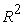and MAE showed how the models performed in prediction of the manually collected biomass. The AIC determined the relative information value of the model using the maximum likelihood estimate and the number of parameters (independent variables) in the model. The formula for AIC is given by;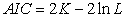where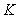is the number of features used and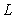is the log-likelihood estimate (the likelihood that the model could have produced the observed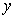-values. The smaller the AIC value meant that the better the model fit.

The BIC is a method for scoring and selecting a model. The BIC statistic was calculated as;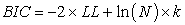LL is the log-likelihood of the model, N is the number of examples in the training dataset, and k is the number of parameters in the model. Based on BIC, more complex models had larger score and in turn, were less likely to be selected.

Root Mean Square Error (RMSE) was the standard deviation of the residuals. Residuals were a measure of how far from the regression line data points are. The RMSE was calculated as;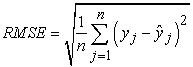A low RMSE value indicated that the simulated and observed data were close to each other showing a better accuracy. Thus lower the RMSE meant that the better the model performance.

Mean Absolute Error (MAE) measured the average magnitude of the errors in a set of predictions, without considering their direction. The MAE values were computed as;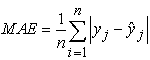A good MAE was relative to a specific dataset. It was a good idea to first establish a baseline MAE for a dataset using a naive predictive model. A model that achieved a MAE better than the MAE for the naive model had a better accuracy.

### 3. Results and Discussion

3.1. Preliminary Analysis

The preliminary analysis involved fitting a linear model between manually measured plant biomass (dry weight) and some selected phenotypic features such as volume, side height and side area. This was to establish if there was any relationship between the manually collected biomass and the plant phenotypic features from the image derived data. From the fitted model, the selected image features were significant predictors of plant biomass [(p < 0.05) Table 2]. The fitted models showed different strengths in predicting the plant biomass (Table 2). The model that was fitted using plant volume and side area showed the best results in terms of adjusted R-squared (Table 2). This showed that the manually collected plant biomass was correlated with the image derived phenotypic features. Additionally, there was also linear relationship between plant biomass and image derived phenotypic features such as plant volume. This suggested that plant biomass could be predicted using the features from the high throughput image derived data. The findings of this current study are in agreement with that of 28 who applied a logistic model for prediction of maize yield under water and nitrogen management and the results showed that the model predicted the maize yield during the growing season with an acceptable accuracy. The findings of this current study are also in agreement with those of 29 who showed that the logistic model is good at estimating the above-ground biomass from the plant height.

• Figure 1. Diagrammatic representation of the linear relationship between manually measured plant dry weight biomass and image derived plant volume phenotypic feature
3.2. Fitted Extreme Gradient Boosting Models

The extreme gradient boosting models were fitted using image derived plant phenotypic features extracted from feature importance (Table 1) at various days after sowing (DAS). The values of the RMSE,and MAE showed how the models performed in prediction of plant biomass from image derived phenotypic features (Table 3). On the basis of the RMSE, the best model was the model that attained the least value of the RMSE [(2.1641) Table 3]. The model was with the least value of RMSE (model at 36 DAS) also had the highest value of(0.8292) (Table 3). Thevalue (0.8292) of the model showed that 82.92% of the variation in plant biomass was accounted for by the used selected image derived phenotypic features. On the basis of the mean absolute error (MAE) metric, the model that had the best performance was the model outputted the least value of the MAE (Table 3). These findings are consistent with those of 23, who evaluated the fitted XGBoost model using R-squared, RMSE, and MAE performance metrics. In their analysis, the fitted XGBoost model delivered the best results 23. Using the R-squared, RMSE, and MAE performance criteria, it was also discovered that the XGBoost model outperformed Artificial Neural Networks and Support Vector Regression 30. 31 compared performance linear model, random forest, support vector regression, XGBoost, LASSO regression, and ensemble technique using R-squared, RMSE, and MAE performance measures, with the XGBoost model yielding the best results.

3.3. Fitted Light Gradient Boosting Models

The light gradient boosting models were fitted using the image derived plant phenotypic features extracted from feature importance (Table 1). From the results the best model on the basis of the RMSE was the model that attained the least value of the RMSE [(2.767341) Table 4]. The values of the RMSE showed how the models performed in prediction of plant biomass from image derived phenotypic features. On the basis of the MAE metric, the model that had the best performance was the model that outputted the least value of the MAE [(MAE=2.012106) Table 4]. This findings are similar to those of 32 Kopitar et al. (2020) who showed that Light GBM outperformed random forest and generalized linear models when their performance was evaluated using RMSE and MAE performance metrics. In a similar study, RMSE and MAE performance criteria showed that the light GBM outperformed the adaBoost model for regression 33. A study by 34 showed that the light GBM outperformed neural networks and decision trees when the performance was evaluated using RMSE and MAE performance criteria.

3.4. Fitted Gompertz Models

The gompertz models were fitted using the image derived plant volume and side area phenotypic features. These are the features that attained the best feature importance score (Table 1). The Gompertz model fitted using the plant volume phenotypic feature had parameters estimates of 3.520762e+08 (8.305924e+07), 1.055582e+02 (2.897641e+01) and 3.348069e-01 [(3.620450e-02) (Table 5). The values in parenthesis represents the standard errors of the estimates. These parameters were significant at 5% level of significance (Table 5). In the model output, the parameters were represented by symbols Asym, b2 and b3. The symbol Asym represented the asymptote or the carrying capacity obtained by doing the limit of the function when volume tends to infinity. This simply showed the highest plant volume that could be attained. The symbol b2 represented the displacement on the x-axis. The symbol b3 was the growth rate. The log likelihood, the AIC, BIC and the deviance statistic values for this model are: -69865.1, 139738.3, 139763.4 and 3.97917e+17, respectively (Table 6). The results showed that the log-likelihood value for the model can range from negative infinity to positive infinity 35. The log-likelihood, AIC and BIC values for a given model are mostly meaningless, but they are useful for comparing two or more models 35.

The Gompertz model fitted using image derived plant side area phenotypic feature had parameters estimates of 7.882705e+06 (2.460224e+06), 2.747763e+0 (2.719820e+00) and 5.416621e-01 (3.035230e-02) and its parameters were significant at 5% level of significance (Table 7). The values in parenthesis represents the standard errors of the estimates. The estimates represented the maximum volume that could be attained using the model, the displacement on the x-axis and the growth rate respectively. The log likelihood, the AIC and BIC values for this model are: -49214.0, 98436.15, 98461.31 and 1.251596e+13, respectively (Table 8). The log likelihood, the AIC and BIC values were used for models comparison. These values were very useful in comparing the different models. The Gompertz growth curves showed that growth is slowest at the start and end of a given time period (Figure 2 & Figure 3).

• Figure 2. Gompertz growth curve generated using image derived plant volume phenotypic feature
• Figure 3. Gompertz growth curve generated using image derived plant side area phenotypic feature

The results showed that plant biomass accumulation in maize followed a sigmoidal growth. The increase is from a fixed point origin (germination point). As the plant develop and produces additional leaves and thus enabling the growth of either more or larger leaves, this creates an acceleration portion of the growth curve. Later in the plant development stage as the plant switch to the reproductive phase, the accumulation of additional plant biomass slows down which ultimately give an S-shaped curve. The dataset for this study did not extend into reproductive phase and thus captured only the first phase of the sigmoidal biomass accumulation plants pattern, hence producing J-shaped curves. This study findings is in agreement with that of 36 who investigated a simple mathematical model that described the growth of the area and the number of total and viable cells in yeast colonies. The study showed that with low inocula, viable cells showed an initial short exponential phase when the colonies were not visible. This phase was shortened with higher inocula. In visible or mature colonies, cell growth displayed Gompertz-type kinetics. It was concluded that the cells growth in colonies was similar to liquid cultures only during the first hours, the rest of the time they grow, with near-zero specific growth rates, at least for 3 weeks 36. 37 studied maize dry matter production and macronutrient extraction model as a new approach for fertilizer rate estimation. The study showed that dry matter accumulation followed a sigmoidal model and the macronutrient content a power model. Biomass accumulation and partitioning in maize has also been found to follow a sigmoidal curve with an exponential phase followed by a linear ending with the senescence phase 38.

39 did comparison of nonlinear models to describe the feather growth and development curve in yellow-feathered chickens. The study showed that the inflection point for the whole-body feather mass occurred, which was interpreted as the age of maximum feather mass gain as indicated by the change in the increase in feather mass from fast to slow 38. The findings of this current study are in agreement with that of 40 who applied Gompertz model to describe the growth of corn. The study showed a slow growth rate at the initial stage, followed by a rapid growth stage to a critical point then the rate of growth began to decline reaching to a stability phase. The findings of this current study are also in agreement with those of 41 who applied Gompertz curve to the growth of tobacco leaves and stem. The study showed that the growth of tobacco leaves and stem was slowest at the start and end of a given time period.

3.5. Fitted Logistic Curve Models

The logistic growth models were fitted using the image derived plant volume and side area phenotypic features. These are the features that attained the best feature importance scores. The model fitted using image derived plant volume phenotypic feature had parameters estimates of 7.049763e+07 (1.642039e+06), 3.281341e+01 (3.418623e-01) and 5.805428e+00 (1.632803e-01) and its parameters were significant at 5% level of significance (Table 9). The values in parenthesis represents the standard errors of the estimates. The estimates represented the maximum volume that could be attained using the model, the displacement on the x-axis and the growth rate respectively. The log likelihood, the AIC and BIC values for this model are: -69870.61, 139749.2, 139774.4 and 3.990138e+17, respectively (Table 10). The log likelihood, the AIC and BIC values for the logistic curve model fitted using image derived plant volume phenotypic feature were used for models comparison. The model fitted using image derived plant side area phenotypic feature had parameters 585775.55336 (9784.1058903), 31.61234 (0.2885569) and 7.01180 (0.1357929) and its parameters were significant at 5% level of significance (Table 11). The values in parenthesis represents the standard errors of the estimates. The estimates represented the maximum plant volume that could be attained using the model, the displacement on the x-axis and the growth rate respectively. The log likelihood, the AIC, BIC and the deviance statistic values for this model are: -49203.98, 98415.95, 98441.11 and 1.245267e+13, respectively (Table 12). The log likelihood, the AIC and BIC values for the logistic curve model fitted using image derived plant side area were used for models comparison The logistic growth curves for models fitted using volume and side area showed that growth is slowest at the start and end of a given time period (Figure 4 & Figure 5).

The findings demonstrated that maize plant biomass accumulation follows a sigmoidal growth pattern. These findings are in agreement with those of 42 who using logistic regression model showed that the plant growth is slowest at the start and end of a given time period. A study by 43 that investigated patterns of dry biomass accumulation and nutrient uptake by okra (Abelmoschus esculentus L.) under different rates of nitrogen application found out that growth followed a sigmoid curve which was accurately described a logistic equation.

• Figure 4. Logistic growth curve fitted using image derived plant volume phenotypic feature
• Figure 5. Logistic growth curve fitted using image derived plant side area phenotypic feature
3.6. Comparison of the Statistical Power of the Models for Plant Growth

The comparison of the statistical power of the models that were used for plant growth, involved comparison of the prediction power of the classical models against the machine leaning models. Further, the two machine learning models were also compared to find out, which had a better prediction power. The comparison metrics that were used in this case were the root mean squared error, R-squared, mean absolute error, Akaike information criterion and Bayesian information criterion. Root mean squared error estimated the accuracy of the forecasting models predicted values versus the actual or observed values while training the regression models. It was used to measure the error in the predicted values since the target or response variable was a continuous number. This criterion was essential in shortlisting the best performing model among different forecasting models that were trained on one particular dataset. The comparison was simply done by comparing the RMSE values across all models and select the one with the lowest value on RMSE (Table 13). The results in Table 13 showed that the extreme gradient boosting model performed better that the light gradient boosting (smaller values of RMSE and MAE in XGBoost than in Light GBM).

On comparison of the Gompertz growth model and the logistic curve model, the two models showed almost the same values of Akaike information criterion and Bayesian information criterion (Table 14). However, these models have a limitation in that they can model only a single phenotypic feature at a time with their independent variable always being time (DAS). A study by 44 that was in line with this study found out that the Gompertz model is the simplest model in the estimation process, while the logistic model is more difficult in the computing process. The Gompertz and logistic models used in the study by 44 had high degree of accuracy with the value of the coefficient of determination of more than 90%. Other Similar findings showed that Akaike's information criteria (AIC) and Bayesian information criterion (BIC) have been used in comparing several growth models 45. The findings of this current study are in line with those of 45 who showed that Gompertz model and the logistic function yielded a better fit since their values of AIC and BIC were low and that there was no an autocorrelation between the residual values. 40 also showed that a simple sigmoid model is preferred as it is easier to interpret the parameters biologically. The logistic model fitted better in describing the plant height growth compared to Gompertz model, as it yielded coefficient of determination more than 99% 40. Based on the model, the absolute growth rate tended to be bell-shaped and right-skewed for logistic and Gompertz, respectively 40.

Statistically it was not possible to directly compare the machine learning models and the classical growth models 46. This is due to the fact that, the growth models can only accommodate one feature at a time with the independent variable always being time intervals 46. However, in this study, it was shown that having a model that fits just one feature is inadequate to predict plant growth because some information is left out. All the features that had been extracted at feature selection stage could all not be accommodated in the Gompertz or the logistic function model. To show that there was loss of information which in turn led to a reduced statistical power, an XGBoost model with all the best selected 31 image derived phenotypic features was compared with the XGBoost model that had the only one feature that had used in the developing the sigmoid models (Table 15). The model with one feature showed poorer performance in terms of RMSE, MAE and R-squared as compared to the model with the 31 features. This is a clear indication that that the XGBoost and Light GBM models are better for modelling plant growth as compared to the mostly used sigmoid models. In agreement with this findings is a study by 47 who compared a Gompertz model against a machine learning model with the latter attaining superior results. 48 also showed that the machine learning models such as neural networks and XGBoost produced better performance in terms of prediction accuracy when compared with the sigmoid models such as the logistic model and the Gompertz model.

On comparison of the machine learning models, the extreme gradient boosting model showed better performance than the light gradient boosting model (Table 13). These results agree with research findings that have shown that Light GBM is faster and more accurate than Cat Boost and XG Boost using variant number of features and records 49. 50 also found out that LightGBM can significantly outperforms XGBoost and Semi Global Matching with Neural networks in terms of computational speed and memory consumption. In a study comparing Gradient Boosting Decision Tree Algorithms for prediction Performance, LightGBM algorithm had the best performance of the three with a balanced combination of accuracy, speed, reliability, and ease of use, followed by XGBoost with the histogram method, and CatBoost came last with slow and inconsistent performance 51. Another study that concurs with this study found out that non-parametric models offer more accurate predictions since they offer a better fit to data than parametric ones 52. Further, non-parametric algorithms provide a good fit for data since they can fit many forms of a function 52.

### 4. Conclusion

In conclusion, thise study found out that the machine learning models performed better that the conventional sigmoid models in explaining plant growth. The extreme gradient boosting model and the light gradient boosting models had the advantage of modelling several phenotypic features at ones as opposed to the classical models that could model only one phenotypic feature at a go. Further, the results also showed that extreme gradient boosting model showed better performance when modelling plant growth as compared to the light gradient boosting model. The developed models could also be used to predict plant biomass at different developmental stages.

### Acknowledgments

The authors acknowledge the Leibniz Institute of Plant Genetics and Crop Plant Research, Gatersleben, Germany for making available the data used in this study. The data was obtained with the support of grants from the German Federal Ministry of Education and Research (BMBF) and performed within the ConFed projects (identification number: 0315461C). The authors declare no conflicts of interest.

### References

  Fourcaud, T., Zhang, X., Stokes, A., Lambers, H., & Körner, C. (2008). Plant growth modelling and applications: the increasing importance of plant architecture in growth models. Annals of Botany, 101(8), 1053-1063. In article View Article  PubMed  Vos, J., Evers, J. B., Buck-Sorlin, G. H., Andrieu, B., Chelle, M., & De Visser, P. H. (2009). Functional–structural plant modelling: a new versatile tool in crop science. Journal of experimental Botany, 61(8), 2101-2115. In article View Article  PubMed  Rahaman, M., Chen, D., Gillani, Z., Klukas, C., & Chen, M. (2015). Advanced phenotyping and phenotype data analysis for the study of plant growth and development. Frontiers in plant science, 6, 619. In article View Article  PubMed  Dold, Christian. (2018). Climate Change Impacts on Corn Phenology and Productivity. 10.5772/intechopen.76933. In article  Chen, D., Neumann, K., Friedel, S., Kilian, B., Chen, M., Altmann, T., & Klukas, C. (2014). Dissecting the phenotypic components of crop plant growth and drought responses based on high-throughput image analysis. The Plant Cell, 26(12), 4636-4655. In article View Article  PubMed  Klukas, C., Chen, D., & Pape, J. M. (2014). Integrated analysis platform: an open-source information system for high-throughput plant phenotyping. Plant physiology, 165(2), 506-518. In article View Article  PubMed  Junker A, Muraya MM, Weigelt-Fischer K, Arana-Ceballos F, Klukas C, Melchinger AE, Meyer RC, Riewe D, Altmann T (2015) Optimizing experimental procedures for quantitative evaluation of crop plant performance in high throughput phenotyping systems. Frontiers in Plant Sciences. 5(770): 1-21. In article View Article  PubMed  Muraya MM, Chu J, Zhao Y, Junker A, Klukas C, Reif JC, Altmann T (2017) Genetic variation of growth dynamics in maize (Zea mays L.) revealed through automated non-invasive phenotyping. The Plant Journal, 89: 366-380. In article View Article  PubMed  Boyd, D., & Crawford, K. (2011,). Six provocations for big data. In A decade in internet time: Symposium on the dynamics of the internet and society. In article  Unnisabegum, A., Hussain, M., & Shaik, M. (2019). Data Mining Techniques for Big Data, Vol. 6, Special Issue. 10.13140/RG.2.2.25408.07686. In article  Blum, A., Hopcroft, J., & Kannan, R. (2020). Foundations of data science. Cambridge University Press. In article View Article  Neumann, K., Klukas, C., Friedel, S., Rischbeck, P., Chen, D., Entzian, A., & Kilian, B. (2015). Dissecting spatiotemporal biomass accumulation in barley under different water regimes using high-throughput image analysis. Plant, cell & environment, 38(10), 1980-1996. In article View Article  PubMed  Karadavut, U., Palta, C., Kokten, K., & Bakoglu, A. (2010). Comparative study on somen on-linear growth models describing leaf growth of maize. Int. J.Agric. Biol. 12, 227-230. In article  Golzarian, M. R., Frick, R. A., Rajendran, K., Berger, B., Roy, S., Tester, M., & Lun, D. S. (2011). Accurate inference of shoot biomass from high-throughput images of cereal plants. Plant methods, 7(1), 1-11. In article View Article  PubMed  Flombaum, P., & Sala, O. E. (2007). A non-destructive and rapid method to estimate biomass and aboveground net primary production in arid environments. Journal of Arid Environments, 69(2), 352-358. In article View Article  Rani, Sushma & Anurag, & Rao, Srinivas. (2019). Study and Analysis of Noise Effect on Big Data Analytics. 8. 5841-5850. In article  Xu, Y., Qiu, Y., & Schnable, J. C. (2018). Functional modeling of plant growth dynamics. The Plant Phenome Journal, 1(1), 1-10. In article View Article  Bratsas, C., Koupidis, K., Salanova, J. M., Giannakopoulos, K., Kaloudis, A., & Aifadopoulou, G. (2020). A Comparison of Machine Learning Methods for the Prediction of Traffic Speed in Urban Places. Sustainability, 12(1), 142. In article View Article  Kong, Y., & Yu, T. (2018). A deep neural network model using random forest to extract feature representation for gene expression data classification. Scientific reports, 8(1), 1-9. In article View Article  PubMed  Putra, F. H. R., Larkin, D., Khanagha, S., & Panza, K. (2019). Paper to be presented at DRUID19 Copenhagen Business School, Copenhagen, Denmark June 19-21, 2019. In article  Zhang, Y. F., Fitch, P., & Thorburn, P. J. (2020). Predicting the Trend of Dissolved Oxygen Based on the kPCA-RNN Model. Water, 12(2), 585. In article View Article  Greenland, S., Senn, S. J., Rothman, K. J., Carlin, J. B., Poole, C., Goodman, S. N., & Altman, D. G. (2016). Statistical tests, P values, confidence intervals, and power: a guide to misinterpretations. European journal of epidemiology, 31(4), 337-350. In article View Article  PubMed  Shahani, N. M., Zheng, X., Liu, C., Hassan, F. U., & Li, P. (2021). Developing an XGBoost Regression Model for Predicting Young’s Modulus of Intact Sedimentary Rocks for the Stability of Surface and Subsurface Structures. Front. Earth Sci, 9, 761990. In article View Article  Teleken, J. T., Galvão, A. C., & Robazza, W. D. S. (2017). Comparing non-linear mathematical models to describe growth of different animals. Acta Scientiarum. Animal Sciences, 39, 73-81. In article View Article  Sprouffske, K., & Wagner, A. (2016). Growth curver: an R package for obtaining interpretable metrics from microbial growth curves. BMC bioinformatics, 17(1), 172. In article View Article  PubMed  Gachoki, Peter, Moses Muraya, and Gladys Njoroge. "Features Selection in Statistical Classification of High Dimensional Image Derived Maize (Zea Mays L.) Phenomic Data." American Journal of Applied Mathematics and Statistics 10, no. 2 (2022): 44-51. In article View Article  Bermingham, J. R. (2003). On exponential growth and mathematical purity: a reply to Bartlett. Population and Environment, 25(1), 71-73. In article View Article  Sepaskhah, A. R., Fahandezh-Saadi, S., & Zand-Parsa, S. (2011). Logistic model application for prediction of maize yield under water and nitrogen management. Agricultural Water Management, 99(1), 51-57. In article View Article  Xiangxiang, W., Quanjiu, W., Jun, F., Lijun, S., & Xinlei, S. (2014). Logistic model analysis of winter wheat growth on China's Loess Plateau. Canadian Journal of Plant Science, 94(8), 1471-1479. In article View Article  Osman, A. I. A., Ahmed, A. N., Chow, M. F., Huang, Y. F., & El-Shafie, A. (2021). Extreme gradient boosting (Xgboost) model to predict the groundwater levels in Selangor Malaysia. Ain Shams Engineering Journal, 12(2), 1545-1556. In article View Article  Huang, J. C., Tsai, Y. C., Wu, P. Y., Lien, Y. H., Chien, C. Y., Kuo, C. F., ... & Kuo, C. H. (2020). Predictive modeling of blood pressure during hemodialysis: A comparison of linear model, random forest, support vector regression, XGBoost, LASSO regression and ensemble method. Computer methods and programs in biomedicine, 195, 105536. In article View Article  PubMed  Kopitar, L., Kocbek, P., Cilar, L., Sheikh, A., & Stiglic, G. (2020). Early detection of type 2 diabetes mellitus using machine learning-based prediction models. Scientific reports, 10(1), 1-12. In article View Article  PubMed  Liang, Y., Wu, J., Wang, W., Cao, Y., Zhong, B., Chen, Z., & Li, Z. (2019, August). Product marketing prediction based on XGboost and LightGBM algorithm. In Proceedings of the 2nd International Conference on Artificial Intelligence and Pattern Recognition (pp. 150-153). In article View Article  Pu, Q., Li, Y., Zhang, H., Yao, H., Zhang, B., Hou, B., & Zhao, L. (2019). Screen efficiency comparisons of decision tree and neural network algorithms in machine learning assisted drug design. Science China Chemistry, 62(4), 506-514. In article View Article  Abdulhafedh, A. (2017). Incorporating the multinomial logistic regression in vehicle crash severity modeling: A detailed overview. Journal of Transportation Technologies, 7(03), 279. In article View Article  Rivas, E. M., Gil de Prado, E., Wrent, P., de Silóniz, M. I., Barreiro, P., Correa, E. C., & Peinado, J. M. (2014). A simple mathematical model that describes the growth of the area and the number of total and viable cells in yeast colonies. Letters in applied microbiology, 59(6), 594-603. In article View Article  PubMed  Martins, K. V., Dourado-Neto, D., Reichardt, K., Favarin, J. L., Sartori, F. F., Felisberto, G., & Mello, S. C. (2017). Maize dry matter production and macronutrient extraction model as a new approach for fertilizer rate estimation. Anais da Academia Brasileira de Ciências, 89, 705-716. In article View Article  PubMed  Connor, D. J., Loomis, R. S., & Cassman, K. G. (2011). Crop ecology: productivity and management in agricultural systems. Cambridge University Press. In article View Article  Xie, W. Y., Pan, N. X., Zeng, H. R., Yan, H. C., Wang, X. Q., & Gao, C. Q. (2020). Comparison of nonlinear models to describe the feather growth and development curve in yellow-feathered chickens. Animal, 14(5), 1005-1013. In article View Article  PubMed  Wardhani, W. S., & Kusumastuti, P. (2014). Describing the height growth of corn using Logistic and Gompertz model. AGRIVITA, Journal of Agricultural Science, 35(3), 237-241. In article View Article  Ismail, Z., Khamis, A., & Jaafar, M. Y. (2003). Fitting nonlinear Gompertz curve to tobacco growth data. Pakistan Journal of Agronomy, 2(4), 223-236. In article View Article  Tessmer, O.L., Jiao, Y., Cruz, J.A., Kramer, D.M., & Chen, J. (2013). Functional approach to high-through put plant growth analysis. BMCSyst.Biol. 7 (Suppl.6): S17. In article View Article  PubMed  Moustakas, N. K., Akoumianakis, K. A., & Passam, H. C. (2011). Patterns of dry biomass accumulation and nutrient uptake by okra ('abelmoschus esculentus'(L.) Moench.) Under different rates of nitrogen application. Australian Journal of Crop Science, 5(8), 993-1000. In article  Noor, R. R., Saefuddin, A., & Talib, C. (2012). Comparison on accuracy of Logistic, Gompertz and Von Bertalanffy models in predicting growth of new born calf until first mating of Holstein Friesian heifers. Journal of Indonesian Tropical Animal Agriculture, 37(3), 151-160. In article View Article  Sariyel, V., Aygun, A., & Keskin, I. (2017). Comparison of growth curve models in partridge. Poultry Science, 96(6), 1635-1640. In article View Article  PubMed  Levy, J., Mussack, D., Brunner, M., Keller, U., Cardoso-Leite, P., & Fischbach, A. (2020). Contrasting classical and machine learning approaches in the estimation of value-added scores in large-scale educational data. Frontiers in psychology, 2190. In article View Article  PubMed  Hansen, B. D., Tamouk, J., Tidmarsh, C. A., Johansen, R., Moeslund, T. B., & Jensen, D. G. (2020, July). Prediction of the Methane Production in Biogas Plants Using a Combined Gompertz and Machine Learning Model. In International Conference on Computational Science and Its Applications (pp. 734-745). Springer, Cham. In article View Article  Ravnik, J., Jovanovac, J., Trupej, A., Vištica, N., & Hriberšek, M. (2021). A sigmoid regression and artificial neural network models for day-ahead natural gas usage forecasting. Cleaner and Responsible Consumption, 3, 100040. In article View Article  AlDaoud, E. (2019). Comparison between XGBoost, LightGBM and CatBoost using a home credit dataset. International Journal of Computer and Information Engineering, 13(1), 6-10. In article  Ke, G., Meng, Q., Finley, T., Wang, T., Chen, W., Ma, W., & Liu, T. Y. (2017). Lightgbm: A highly efficient gradient boosting decision tree. Advances in neural information processing systems, 30. In article  Alshari, Haithm & Saleh, Yahya & Odabas, Alper. (2021). Comparison of Gradient Boosting Decision Tree Algorithms for CPU Performance. Erciyes Tip Dergisi. 157-168. In article  Marques, Armando & Prates, Pedro & Pereira, André & Oliveira, M. & Fernandes, José & Ribeiro, Bernardete. (2020). Performance Comparison of Parametric and Non- Parametric Regression Models for Uncertainty Analysis of Sheet Metal Forming Processes. Metals. In article View Article

Published with license by Science and Education Publishing, Copyright © 2022 Peter Gachoki, Moses Muraya and Gladys NjorogeThis work is licensed under a Creative Commons Attribution 4.0 International License. To view a copy of this license, visit http://creativecommons.org/licenses/by/4.0/